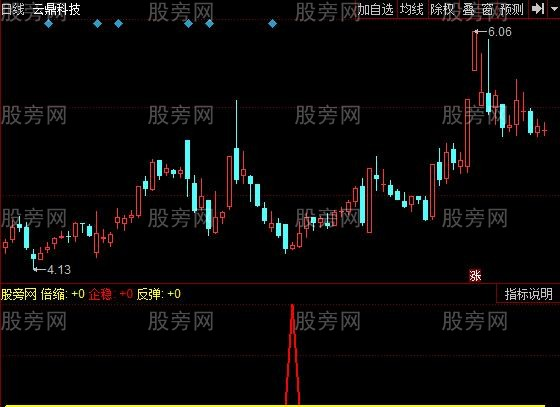# 同花顺瞬秒主力指标公式

XA_1:=(CLOSE-REF(CLOSE,1))/REF(CLOSE,1)>=0.04 OR CLOSE/OPEN>=1.03 AND (vol<=ma(VOL,60) OR VOL/REF(VOL,1)<=1.2 OR VOL<HHV(VOL,5)) AND HIGH>LOW;
XA_2:=(OPEN-CLOSE)/OPEN>=0.04 AND (VOL/MA(VOL,60)<=2/3 OR VOL/REF(VOL,1)<=1.15 OR VOL<HHV(VOL,5));
XA_3:=VOL/REF(VOL,1)<=0.5 AND REF(VOL,2)>REF(VOL,1) AND REF(CLOSE,1)<REF(OPEN,1) AND CLOSE<OPEN;
XA_4:=REF(XA_2,1) AND VOL/REF(VOL,1)<=0.52;
XA_5:=REF(XA_2,1) AND VOL/REF(VOL,1)<=0.52 AND CLOSE>OPEN;

stICKLINE(倍缩 AND NOT(DATE=1160107),0.37,0,5,0),coloryellow;
XA_6:=VOL>REF(VOL,1)*0.5 AND CLOSE>OPEN;
XA_7:=COUNT(倍缩,5) AND (XA_6 OR XA_5 OR REF(XA_5,1)) AND (REF(VOL,1)/REF(VOL,2)<=1.98 OR REF(XA_2,1));### 相关文章